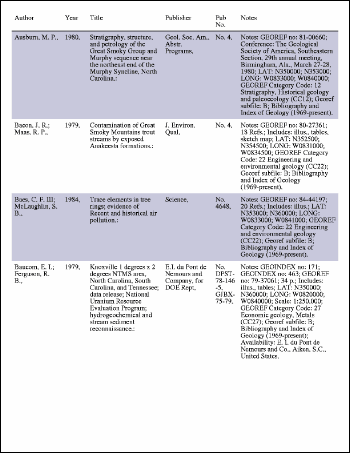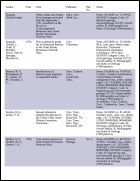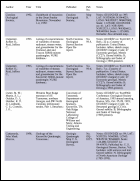Large Table ExampleThis example shows how to draw a multi-page table. ABCpdf does not provide table drawing routines itself so this example uses a Table Class to position the table elements.Setup

 We start by creating our document object and reading the data for our table. For the purposes of this example, we'll assume that our data is in a standard tab delimited format. ```'... read the text Set fso = CreateObject("Scripting.FileSystemObject") Set f = fso.OpenTextFile(Server.MapPath("text7.txt"), 1) theText = f.ReadAll() Set theDoc = Server.CreateObject("ABCpdf12.Doc") ' set up document theDoc.FontSize = 12 theDoc.Rect.Inset 20, 20```Rotate

 We create a new table object and focus on our document. Focusing tells the table what rectangle it can occupy (it takes the current document rectangle) and how many columns of data it should be prepared for. Columns are assigned relative widths and expand horizontally to fit the table rectangle. Here, we're specifying six columns and a number of relative widths. Columns one and four are not assigned a width so they default to a relative width of one. Finally, we're padding the cells so there are gaps between the rows and columns. ```Set theTable = New Table ' focus the table on the document theTable.Focus theDoc, 6 ' some columns extra width theTable.Width(0) = 2 theTable.Width(2) = 3 theTable.Width(3) = 2 theTable.Width(5) = 4 ' ensure columns are padded theTable.Padding = 5```We iterate through the table data adding rows and columns as we go. Every time we add a row, we check to see whether we've reached the end of the page. When we get there, we check the last row we added to ensure that it wasn't truncated. If it was, then we delete it and prepare to insert it again. We add a new page, prepare to draw the table headers again and reset the row framing and row shading parameters. We insert all our content into the current row, cell by cell. After we've inserted all our content into the row, we select it and add a frame or background shading as appropriate. Finally, we save the document. ```theFrame = True theShade = False theRows = Split(theText, vbCrLf) i = 0 Do   ' add in new row - see if it fitted onto page   If theTable.NextRow = False Then     ' delete and draw last row again?     If theTable.RowTruncated Then       theTable.DeleteLastRow       i = i - 1     End If     ' insert headers     i = i - 1     If i >= 0 Then theRows(i) = theRows(0)     ' frame row and reset shading     theFrame = True     theShade = False     ' add new page to table     theDoc.Flatten     theTable.NewPage   End If   ' get next row   If i < 0 Or i > UBound(theRows) Then Exit Do   theRow = theRows(i)   i = i + 1   ' add all the cells into a row   theCols = Split(theRow, vbTab)   For j = 0 To UBound(theCols)     theTable.NextCell     theTable.AddText theCols(j)   Next   ' underline first row & shade every other row   theTable.SelectRow theTable.Row   If theFrame = True Then theTable.Frame False, True, False, False   If theShade Then theTable.Fill "200 200 220"   theFrame = False   theShade = Not theShade Loop theDoc.Flatten theDoc.Save "c:\mypdfs\table2.pdf"```Results

Using a large quantity of input data, we get the following output.table2.pdf [Page 1]table2.pdf [Page 2]table2.pdf [Page 3]table2.pdf [Page 4]table2.pdf [Page 5]